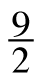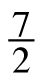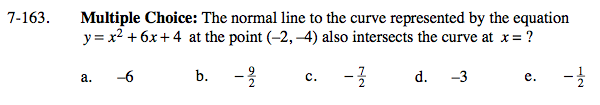### Home > CALC > Chapter 7 > Lesson 7.3.5 > Problem7-163

7-163.
1. Multiple Choice: The normal line to the curve represented by the equation y = x2 + 6x + 4 at the point (−2, −4) also intersects the curve at x = ? Homework Help ✎

1. −6

2.3.4. −3

5.A normal line is perpendicular to the tangent line, at the point of tangency.
So if you can find the tangent line, then you can also find the normal line.

Let the equation of the normal line = x² + 6x + 4 and find the TWO points of intersection.